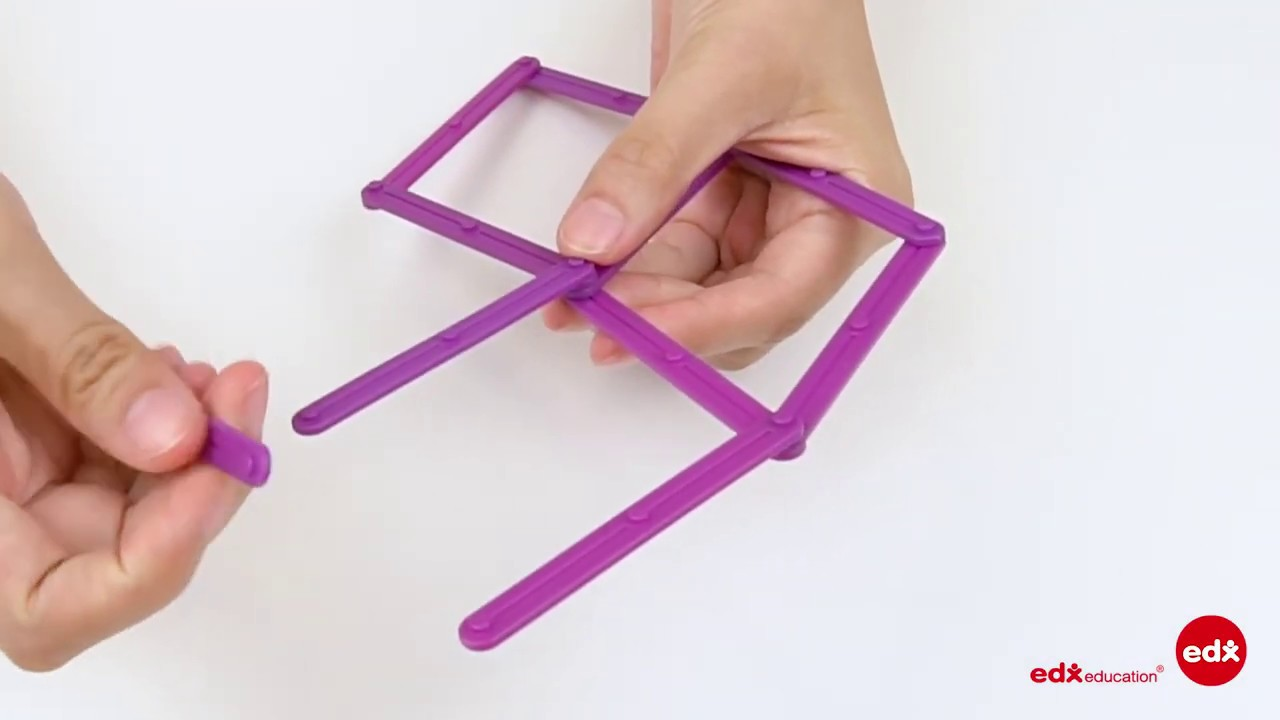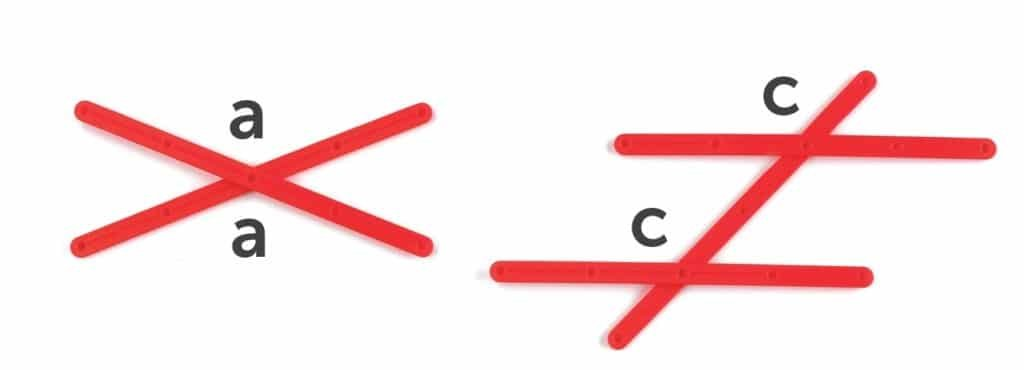# Geostix

#### • Measurement: Angle

GeoStix are an ideal construction tool. Students can make a variety of 2D shapes by  connecting various length sticks together. The sticks come in 10 colours and 8 different lengths. There is a quarter circle and half circle. This allows students to explore many geometry and measurement concepts.

### GeoStix Videos### Mathematical Language

While using GeoStix students will be using measurement language, eg long, longer, longest, short, shorter, shortest. They will use geometric language such as the names of shapes, eg triangle: equilateral (all sides the same length), isosceles (two sides the same length) and scalene (all sides different lengths).

### Using Geostix

Geostix are a versatile manipulative. They may be used by young children to construct shapes. Later Geostix may be used to formalise many geometric ideas. Examples of this are noted in the Construction Triangles section.

#### Construction

Students may create many different constructions from informal to more formal shapes such as triangles, squares and polygons. See the Exploring Properties of Quadrilaterals section for examples.

#### Angle Relationships

Later, constructions can become more directed so that specific relationships may be noted. For example, joining two GeoStix
together allows students to explore vertically opposite angles. Encourage children to explore other angle types by joining GeoStix in parallel lines.Vertically Opposite

Corresponding

In the triangle explorations students learned about triangles. Geostix may be used to explore properties of other polygons such as quadrilaterals. Diagonals may be shown using Geostix.